# Math Practice Topic: Irregular Shape Areas

 Description: This topic deals with figuring out the area of irregular shapes by breaking down shapes. These problems are difficult and not intended for most students. However, this topic might serve as useful training for the SAT. Adaptive Learning Progression: Problems become harder. Start using MathScore for free

## Sample Levels (out of 3)

Find the area of the highlighted region. Gives answers to the nearest hundreth. Use 3.14 for pi

 1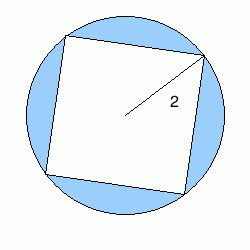The figure inside is a square. Area: 2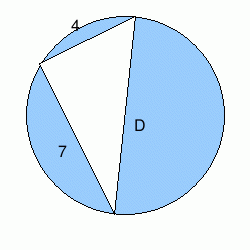The triangle inside is a right triangle. Area:

Find the area of the highlighted region. Gives answers to the nearest hundreth. Use 3.14 for pi

 1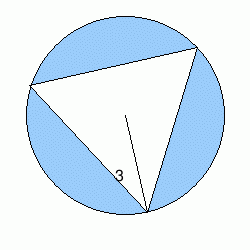The triangle inside is equilateral. Area: 2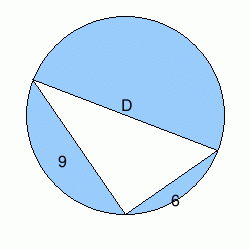The triangle inside is a right triangle. Area: 3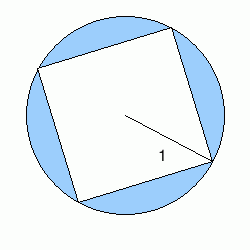The figure inside is a square. Area: 4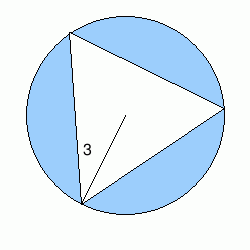The triangle inside is equilateral. Area: